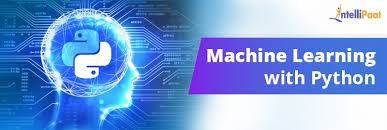# A Beginner's Guide To Machine Learning

Artificial Intelligence (AI) is a way to simulate human intelligence in machines. AI is like creating babies out of machines. Just like how you teach a baby to everything from scratch, you also have to teach machines everything from scratch. The only difference is that babies have brains that are responsible for processing all the information they absorb through their surroundings. But machines cannot do that. For machines, we have to create algorithms that can try to mimic the functionalities of the human brain. This is where Machine Learning (ML) comes into the picture. ML is one way to realize Artificial Intelligence.

Machine Learning as defined by Wikipedia is, “ Machine learning (ML) is the scientific study of algorithms and statistical models that computer systems use to perform a specific task without using explicit instructions, relying on patterns and inference instead. It is seen as a subset of artificial intelligence. Machine learning algorithms build a mathematical model based on sample data, known as 'training data', in order to make predictions or decisions without being explicitly programmed to perform the task.”

As it is evident from the definition, ML is about algorithms that are designed to carry out tasks like, analyzing high volume data, making predictions based on data, automating mundane tasks, etc. There are various approaches to machine learning which are discussed next.

Thus, the evaluation of students on the basis of machine learning training and explicitly based on them is criteria which the top companies actually put in the portfolio in order to recruit students.

The following are the methods to achieve ML:

Supervised Learning

Unsupervised Learning

Semi-supervised Learning

Reinforcement Learning

Supervised Learning

Supervised Learning is applied if the dataset we have is labelled. It matches input to an output based on input and output pairs. For example, if we want to recognise handwritten digits, the data will have correctly labelled images of handwritten digits. After applying the necessary algorithm, the ML model will be equipped to predict digits written by any person when given as input. There are two ways to approach Supervised Learning:

Regression

Classification

Regression:

In regression, we predict continuous values. It is used to estimate value like housing prices, human lifespan, salary prediction etc. Depending upon the type of problem to be solved, various regression techniques are available like, Linear Regression, Logistic Regression, Polynomial Regression, Ridge Regression etc.

Here, I will explain Linear Regression to give you an idea of how regression works.

Linear regression is used for predictive analysis. This model is made up of linear variables. It models the relationship between single input independent variable and a single output dependent variable using best fit line.

Best fit line is a line that is obtained using the most common mathematical equation: equation of line.

y=a_1*x_1+a_2*x_2+a_1*x_3+⋯+a_n*x_n+b

Here, an are coefficients and xn are variables and b is bias. In it we just realise the importance of each feature and make a prediction.

Classification

In classification, we classify the input to a class based on the training data given to it. There are various types of classifiers used like, linear classifier, Naïve Bayes, SVM, Neural Networks, and Decision Trees etc.

Here we will discuss the basic definition of Logistic Classifier.

Logistic classifier is a statistical method for analysing a data set in which there are one or more independent variables that determine an outcome. The outcome is measured with a dichotomous variable (in which there are only two possible outcomes). The goal of logistic regression is to find the best fitting model to describe the relationship between the dichotomous characteristic of interest (dependent variable = response or outcome variable) and a set of independent (predictor or explanatory) variables. This is better than other binary classification like nearest neighbor since it also explains quantitatively the factors that lead to classification. Also, to know more about machine learning algorithms, one needs to study machine learning tutorial as well.

Unsupervised Learning

In this the models are trained using data that is not labelled or classified. It is up to us to understand the similarities in the data. Unsupervised learning classified into two categories of algorithms:

Clustering: A clustering problem is where you want to discover the inherent groupings in the data, such as grouping customers by purchasing behaviour.

Association: An association rule learning problem is where you want to discover rules that describe large portions of your data, such as people that buy X also tend to buy Y.

Last updated:11/27/2019 4:23:10 AM#### Jimmy Reece

Professional Developer and Procurator of Machine Learning Training In Bangalore